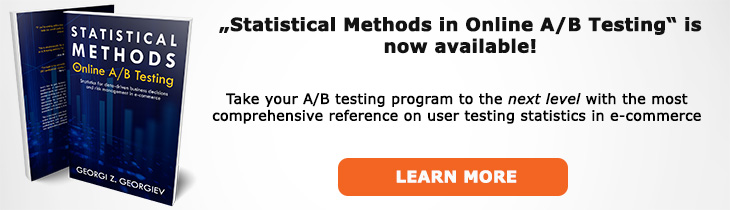# What does "beta" mean?

Definition of beta in the context of A/B testing (online controlled experiments).

## What is beta?

The greek leter beta (β) is most-often used to denote the pre-test probability of committing a type II error deemed satisfactory for the test at hand given a specific effect of interest. A test with a lower β is more sensitive towards smaller departures from the null hypothesis and thus has a greater probability of rejecting a false null.

Beta is inversely related to statistical power since POW = 1 - β (from POW(T(α); μ1) = P(d(X) > c(α); μ1) and β(T(α); μ1) = P(d(X) ≤ c(α); μ = μ1) for any μ1 greater than μ0, where c(α) is the significance threshold, d(X) is a test statistic (distance function), μ is the true magnitude of the effect while μ1 is the magnitude of the effect under a particular alternative hypothesis H1).

After a test is complete beta somewhat loses its purpose and while it can still be computed versus different values of interest using a confidence interval to judge the test's sensitivity based on the interval width is a more compelling approach. It should be noted that in case the result was just significant, the test\'s power is always equal to 0.5.

The error rates alpha (α) and beta (β) are inversely related: increasing one decreases the other, assuming fixed variance, sample size and minimum effect of interest.

## Related A/B Testing terms

Statistical Power

Type II Error

## Articles on beta

Like this glossary entry? For an in-depth and comprehensive reading on A/B testing stats, check out the book "Statistical Methods in Online A/B Testing" by the author of this glossary, Georgi Georgiev.## Glossary Index by Letter

Select a letter to see all A/B testing terms starting with that letter or visit the Glossary homepage to see all.